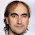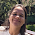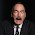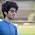Sunday, June 9, 2013

Leap Motion Controller and JavaFX: A new touch-less approach

Hi, it's been a while since my last post, but this first half of the year I've been quite busy at work. So I had to put on hold most of my JavaFX, Raspberry Pi and Arduino projects.

In all this time I could (even) afford only one distraction, because the device really deserve it!

In April I had the chance to get involved in the Leap Motion Developer program (thanks for that to Jim Weaver and Simon Ritter, and of course, to the Leap Motion staff), and since I received the little but powerful device at home, I've been playing around with it in several JavaFX based projects.

So this post is a little briefing of the few projects I've done with the Leap Motion controller and JavaFX, most of them just as a Proof of Concept.

As the Leap SDK is private for now (though they intend to make it public soon), I won't release any code, just snippets, few screenshots and short videos.

At a glance, this is what I'll cover:
• The Leap Motion Controller, what you can expect: hands, fingers, tools and basic gestures.
• The JavaFX and the Leap threads, change listeners to the rescue.
• POC #1. Moving 2D shapes on a JavaFX scene, trapping basic gestures with the Leap.
• POC #2. Physics 2D worlds and Leap, a JavaFX approach.
• POC #3. JavaFX 3D and Leap, with JDK8 early preview and openJFX Project.
I won't go in much detail regarding the Leap itself. There're plenty of videos out there. If you don't know about it yet, check these, for instance:
For those of you already on the pre-order list, the 22nd of July it's already there... be (just a little bit more) patient! For those who haven't decided to buy one yet, maybe this reading will help you make up your mind.

Let's go!

1. The Leap Motion Controller

After you plug in the Leap Motion device in your USB port (Windows, Mac and Linux OS), and download and install its sofware, you can try the Leap Visualizer, a bundled application which allows you to learn and discover the magic of the Leap.

As soon as you launch it, you can virtually see your hands and fingers moving around the screen. It's really impressive because of the high precision of the movements due to the high frequency in which the Leap scans.

Activating hands and fingers visualization you can realize those are the basics of the model provided: the Leap will detect none, one or several hands, and several fingers in each one of them. For each hand, it will show, for instance, its position, where it points at (hand direction) and its palm normal. For fingers, you'll get their position and where they point at. Also you can get hand or fingers velocity.

These positions, directions and velocities of your real hands and fingers are 3D vectors, refered to a right-handed Cartesian coordinate system, with the origin at the center of the device and the X and Z axes lying in the horizontal plane and the Y axis is vertical.

It's important to notice you'll have to convert these coordinates to the ones of the screen if you want to display and move anything on it. For that, you need to calculate where the vector of the hand or finger direction intersects with the plane of the screen.

The Leap device performs complete scans of its surroundings, with an effective range approximately from 25 to 600 millimeters above the device (1 inch to 2 feet). Each scan defines a frame, with all the data associated.

The scan rate is really high, that's what makes the Leap so impressive and accurate compared to similar devices. Depending on your CPU and the amount of data analyzed, the range of processing latency goes from 2 ms to 33 ms, giving rates from 30 to 500 fps.

Besides directions, a basic collection of gestures is also provided: key tap or screen tap, swipe and circle gestures are tracked by comparing the finger movements through different frames.

The good thing of having access to all frames data is that you can define your custom gestures, and try to find them analyzing a relative short collection of frames, all over again.

To end this brief intro to the great Leap Motion device, let's say that being on the Developer Program you can get your SDK for many programming languages, such as Java, JavaScript, C++, C#, Objetive C or Phyton.

In terms of Java code, all you need to do is extend the Listener class provided by the SDK and basically override the onFrame method, and let the magic begin.

2. The JavaFX and the Leap threads

Having a Leap Motion Controller means you can interact with your applications in a very different way you're used to. For that, you just need to integrate the Leap events, in terms of movement or actions, in your apps.

In a JavaFX based application, one easy way to do this is by adding ObjectProperty<T> objects to the LeapListener class in order to set desired values at every frame using Vector, Point2D, Point3D, CircleGesture,... and then implement their related public ObservableValue<T> methods.

Then, in the JavaFX thread, an anonimous ChangeListener<T> class can be added to listen for any change in the ObservableValue. Special care must be taken here, as anything related to the UI must be deal by Platform.runLater().

The next proof of concept samples will try to explain this.

3. POC #1. Moving 2D shapes on a JavaFX scene

Let's say we want to move a node in the scene with our hand as a first simple POC.

We create two classes: SimpleLeapListener class, that extends Listener, where we just set at every frame the coordinates of the screen where the hand points at:

public class SimpleLeapListener extends Listener {

private ObjectProperty<Point2D> point=new SimpleObjectProperty<>();

public ObservableValue<Point2D> pointProperty(){ return point; }

@Override
public void onFrame(Controller controller) {
Frame frame = controller.frame();
if (!frame.hands().empty()) {
Screen screen = controller.calibratedScreens().get(0);
if (screen != null && screen.isValid()){
Hand hand = frame.hands().get(0);
if(hand.isValid()){
Vector intersect = screen.intersect(hand.palmPosition(),hand.direction(), true);
point.setValue(new Point2D(screen.widthPixels()*Math.min(1d,Math.max(0d,intersect.getX())),
screen.heightPixels()*Math.min(1d,Math.max(0d,(1d-intersect.getY())))));
}
}
}
}
}

And LeapJavaFX, our JavaFX class, that listen to changes in this point and reflect them on the scene:

public class LeapJavaFX extends Application {
private SimpleLeapListener listener = new SimpleLeapListener();
private Controller leapController = new Controller();

private AnchorPane root = new AnchorPane();
private Circle circle=new Circle(50,Color.DEEPSKYBLUE);

@Override
public void start(Stage primaryStage) {

final Scene scene = new Scene(root, 800, 600);

@Override
public void changed(ObservableValue ov, Point2D t, final Point2D t1) {
Platform.runLater(new Runnable(){
@Override
public void run() {
Point2D d=root.sceneToLocal(t1.getX()-scene.getX()-scene.getWindow().getX(),
t1.getY()-scene.getY()-scene.getWindow().getY());
double dx=d.getX(), dy=d.getY();
if(dx>=0d && dx<=root.getWidth()-2d*circle.getRadius() &&
circle.setTranslateX(dx);
circle.setTranslateY(dy);
}
}
});
}
});

primaryStage.setScene(scene);
primaryStage.show();
}
@Override
public void stop(){
leapController.removeListener(listener);

}
}

Pretty simple, isn't it? This short video shows the result.

Here goes a second sample, based on the same idea, one circle per detected hand it's displayed, with its radius growing or shrinking according the Z distance of the hand to the Leap. When key tap kind of gestures are detected, a shadow circle is shown where the tap occurs, moved from the previous tap location with an animation of the changes in both transition and scale properties.

Here you can see it in action:

4. POC #2. Physics 2D worlds and Leap, a JavaFX approach

When Toni Epple saw this video, he suggested me to add some physics to the mix, so I started learning from his blog posts about JavaFX and JBox2D, the Java port of the popular Box2D physics engine. Using his amazing work I was able to create a simple World, add some dynamic bodies and static walls to the boundaries, and a static big circle which I could move with the Leap, as in the previous samples. Thank you, Toni, your work is really inspiring!

Here is a code snippet of the JavaFX class.

public class PhysicsLeapJavaFX extends Application {
private SimpleLeapListener listener = new SimpleLeapListener();
private Controller leapController = new Controller();

private Button button=new Button("Add Ball");
private AnchorPane root = new AnchorPane();
private AnchorPane pane = new AnchorPane();
private Body myCircle=null;

private World world=null;
private WorldView worldView=null;
private final float worldScale=50f;
private final float originX=4f, originY=8f;
private final float radius=1f;

@Override
public void start(Stage primaryStage) {

world = new World(new Vec2(0, 0f)); // No gravity
// 200x400 -> world origin->(4f, 8f), Y axis>0 UP
worldView=new WorldView(world, originX*worldScale, originY*worldScale, worldScale);

AnchorPane.setBottomAnchor(pane, 20d); AnchorPane.setTopAnchor(pane, 50d);
AnchorPane.setLeftAnchor(pane, 20d);   AnchorPane.setRightAnchor(pane, 20d);
// root: 800x600, pane: 760x530, worldScale= 50 -> world dimensions: 15.2f x 10.6f
pane.getChildren().setAll(worldView);

button.setLayoutX(30); button.setLayoutY(15);
button.setOnAction(new EventHandler<ActionEvent>(){
@Override
public void handle(ActionEvent t) {
Body ball=new CircleShapeBuilder(world).userData("ball")
.position(0f, 4f)
.type(BodyType.DYNAMIC).restitution(1f).density(0.4f)
.build();
ball.setLinearVelocity(new Vec2(4,2));
ball.setLinearDamping(0f);
}
});

myCircle=new CircleShapeBuilder(world).userData("hand1").position(0f, 2f)
.type(BodyType.STATIC).restitution(1f).density(1)
.build();
new BoxBuilder(world).position(3.6f, 8f).restitution(1f).friction(0f)
.halfHeight(0.1f).halfWidth(7.7f).build();
new BoxBuilder(world).position(3.6f, -2.6f).restitution(1f).friction(0f)
.halfHeight(0.1f).halfWidth(7.7f).build();
new BoxBuilder(world).position(-4f, 2.7f).restitution(1f).friction(0f)
.halfHeight(5.4f).halfWidth(0.1f).build();
new BoxBuilder(world).position(11.2f, 2.7f).restitution(1f).friction(0f)
.halfHeight(5.4f).halfWidth(0.1f).build();

final Scene scene = new Scene(root, 800, 600);

@Override
public void changed(ObservableValue<? extends Point2D> ov, Point2D t, final Point2D t1) {
Platform.runLater(new Runnable(){
@Override
public void run() {
Point2D d=pane.sceneToLocal(t1.getX()-scene.getX()-scene.getWindow().getX()-root.getLayoutX(),
t1.getY()-scene.getY()-scene.getWindow().getY()-root.getLayoutY());
double dx=d.getX()/worldScale, dy=d.getY()/worldScale;
if(dx>=0.1 && dx<=pane.getWidth()/worldScale-2d*radius-0.1 &&
myCircle.getAngle());
}
}
});
}
});
@Override public void changed(ObservableValue<? extends Boolean> ov, Boolean t, final Boolean t1) {
if(t1.booleanValue()){
Platform.runLater(new Runnable(){
@Override public void run() {
button.fire();
}
});
}
}
});

primaryStage.setTitle("PhysicsLeapJavaFX Sample");
primaryStage.setScene(scene);
primaryStage.show();
}
@Override
public void stop(){
leapController.removeListener(listener);

}
}

I've added some gesture recognition to fire the button when a key tap gesture it's done. Besides, it's quite convinient to smooth the readings from the Leap, taking the average of the last positions instead of the position for every frame. So let's modify the SimpleLeapListener class adding a size limited LinkedList collection to store the last 30 positions, and also enable the key tap gestures:

public class SimpleLeapListener extends Listener {

private ObjectProperty<Point2D> point=new SimpleObjectProperty<>();
public ObservableValue<Point2D> pointProperty(){ return point; }
private LimitQueue<Vector> positionAverage = new LimitQueue<Vector>(30);

private BooleanProperty keyTap= new SimpleBooleanProperty(false);
public BooleanProperty keyTapProperty() { return keyTap; }

@Override
public void onFrame(Controller controller) {
Frame frame = controller.frame();
if (!frame.hands().empty()) {
Screen screen = controller.calibratedScreens().get(0);
if (screen != null && screen.isValid()){
Hand hand = frame.hands().get(0);
if(hand.isValid()){
Vector intersect = screen.intersect(hand.palmPosition(),hand.direction(), true);
Vector avIntersect=Average(positionAverage);
point.setValue(new Point2D(screen.widthPixels()*Math.min(1d,Math.max(0d,avIntersect.getX())),
screen.heightPixels()*Math.min(1d,Math.max(0d,(1d-avIntersect.getY())))));
}
}
}
keyTap.set(false);
GestureList gestures = frame.gestures();
for (int i = 0; i < gestures.count(); i++) {
if(gestures.get(i).type()==Gesture.Type.TYPE_KEY_TAP){
keyTap.set(true); break;
}
}
}

private Vector Average(LimitQueue<Vector> vectors)
{
float vx=0f, vy=0f, vz=0f;
for(Vector v:vectors){
vx=vx+v.getX(); vy=vy+v.getY(); vz=vz+v.getZ();
}
return new Vector(vx/vectors.size(), vy/vectors.size(), vz/vectors.size());
}

private class LimitQueue<E> extends LinkedList<E> {
private int limit;
public LimitQueue(int limit) {
this.limit = limit;
}

@Override
public boolean add(E o) {
while (size() > limit) { super.remove(); }
return true;
}
}
}

And finally, here you can see it in action:

5. POC #3. JavaFX 3D and Leap, with JDK8

The last part of this post will cover my experiments with the recent early access releases of JDK8, build b92 on the time of this writing, as JavaFX 3D is enabled since b77. Here you can read about the 3D features planned for JavaFX 8.

Installing JDK8 is easy, and so is creating a JavaFX scene with 3D primitives like spheres, boxes or cylinders, or even user-defined shapes by meshes, defined by a set of points, texture coordinates and faces.

There are no loaders for existing 3D file formats (obj, stl, Maya, 3D Studio, ...). So if you want to import a 3D model, you need one.

The first place to start looking is in OpenJFX, the open source code home of JavaFX development.

You'll find in their repository between their experiments, as they call them, a 3D Viewer. So download it from their repository, build it and see what you can do!

For instance, you can drag and drop an obj model. The one in the picture is a model of a Raspberry Pi, downloaded from here.

For other formats not yet supported, you can go to InteractiveMesh.org, where August Lammersdorf has released several importers (3ds, obj and stl) for JDK8 b91+. Kudos to him for his amazing work and contributions!

I'll use his 3ds importer and the Hubble Space Telescope model from NASA, to add this  model to a JavaFX scene, and then I'll try to add touch-less rotation and scaling options.

First of all, we need a little mathematical background here, as rotating a 3D model in JavaFX requires a rotation axis and angle. If we have several rotations to make at the same time we need to construct a rotation matrix, and after that get the rotation axis and its angle.

As the Leap provides three rotations from a hand: pitch (around its X axis) , yaw (around its Y axis) and roll (around its Z axis), providing the model is already well orientated (otherwise we'll need to add previous rotations too), the rotation matrix will be:

where:
So:
Then, the angle and the rotation unitary axis components can be easily computed from:

Special care has to be taken when converting Leap roll, pitch and yaw angles values to those required for the JavaFX coordinate system (180º rotated from X axis).

With this equations, we just need to listen to hand rotation changes and compute the rotation axis and angle values on every change to rotate accordingly the 3D model.

So now we're ready to try our POC 3D sample: import a 3ds model and perform rotations with our hand through the Leap Motion Controller.

The following code snippet shows how it is done for the JavaFX class:

public class JavaFX8 extends Application {
private AnchorPane root=new AnchorPane();
private final Rotate cameraXRotate = new Rotate(0,0,0,0,Rotate.X_AXIS);
private final Rotate cameraYRotate = new Rotate(0,0,0,0,Rotate.Y_AXIS);
private final Translate cameraPosition = new Translate(-300,-550,-700);
private SimpleLeapListener listener = new SimpleLeapListener();
private Controller leapController = new Controller();

@Override
public void start(Stage stage){
final Scene scene = new Scene(root, 1024, 800, true);
final Camera camera = new PerspectiveCamera();
scene.setCamera(camera);

TdsModelImporter model=new TdsModelImporter();
try {
URL hubbleUrl = this.getClass().getResource("hst.3ds");
}
catch (ImportException e) {
System.out.println("Error importing 3ds model: "+e.getMessage());
return;
}
final Node[] hubbleMesh = model.getImport();
model.close();
final Group model3D = new Group(hubbleMesh);

final PointLight pointLight = new PointLight(Color.ANTIQUEWHITE);
pointLight.setTranslateX(800);
pointLight.setTranslateY(-800);
pointLight.setTranslateZ(-1000);

@Override public void changed(ObservableValue<? extends Point3D> ov, Point3D t, final Point3D t1) {
Platform.runLater(new Runnable(){
@Override public void run() {
if(t1!=null){
double roll=listener.rollLeftProperty().get();
double pitch=-listener.pitchLeftProperty().get();
double yaw=-listener.yawLeftProperty().get();
matrixRotateNode(model3D,roll,pitch,yaw);
}
}
});
}
});
}
private void matrixRotateNode(Node n, double alf, double bet, double gam){
double A11=Math.cos(alf)*Math.cos(gam);
double A12=Math.cos(bet)*Math.sin(alf)+Math.cos(alf)*Math.sin(bet)*Math.sin(gam);
double A13=Math.sin(alf)*Math.sin(bet)-Math.cos(alf)*Math.cos(bet)*Math.sin(gam);
double A21=-Math.cos(gam)*Math.sin(alf);
double A22=Math.cos(alf)*Math.cos(bet)-Math.sin(alf)*Math.sin(bet)*Math.sin(gam);
double A23=Math.cos(alf)*Math.sin(bet)+Math.cos(bet)*Math.sin(alf)*Math.sin(gam);
double A31=Math.sin(gam);
double A32=-Math.cos(gam)*Math.sin(bet);
double A33=Math.cos(bet)*Math.cos(gam);

double d = Math.acos((A11+A22+A33-1d)/2d);
if(d!=0d){
double den=2d*Math.sin(d);
Point3D p= new Point3D((A32-A23)/den,(A13-A31)/den,(A21-A12)/den);
n.setRotationAxis(p);
n.setRotate(Math.toDegrees(d));
}
}
}

And this code snippet shows how it is done for the Leap Listener class:

public class SimpleLeapListener extends Listener {
private ObjectProperty<Point3D> posHandLeft=new SimpleObjectProperty<Point3D>();
private DoubleProperty pitchLeft=new SimpleDoubleProperty(0d);
private DoubleProperty rollLeft=new SimpleDoubleProperty(0d);
private DoubleProperty yawLeft=new SimpleDoubleProperty(0d);
private LimitQueue<Vector> posLeftAverage = new LimitQueue<Vector>(30);
private LimitQueue<Double> pitchLeftAverage = new LimitQueue<Double>(30);
private LimitQueue<Double> rollLeftAverage = new LimitQueue<Double>(30);
private LimitQueue<Double> yawLeftAverage = new LimitQueue<Double>(30);

public ObservableValue<Point3D> posHandLeftProperty(){ return posHandLeft; }
public DoubleProperty yawLeftProperty(){ return yawLeft; }
public DoubleProperty pitchLeftProperty(){ return pitchLeft; }
public DoubleProperty rollLeftProperty(){ return rollLeft; }

@Override
public void onFrame(Controller controller) {
Frame frame = controller.frame();
if (!frame.hands().empty()) {
Screen screen = controller.calibratedScreens().get(0);
if (screen != null && screen.isValid()){
Hand hand = frame.hands().get(0);
if(hand.isValid()){
pitchLeft.set(dAverage(pitchLeftAverage).doubleValue());
rollLeft.set(dAverage(rollLeftAverage).doubleValue());
yawLeft.set(dAverage(yawLeftAverage).doubleValue());

Vector intersect = screen.intersect(hand.palmPosition(),hand.direction(), true);
Vector avIntersect=Average(posLeftAverage);
posHandLeft.setValue(new Point3D(screen.widthPixels()*Math.min(1d,Math.max(0d,avIntersect.getX())),
screen.heightPixels()*Math.min(1d,Math.max(0d,(1d-avIntersect.getY()))),
hand.palmPosition().getZ()));
}
}
}
}
private Double dAverage(LimitQueue<Double> vectors){
double vx=0;
for(Double d:vectors){
vx=vx+d.doubleValue();
}
return new Double(vx/vectors.size());
}
}

In the following video I've added a few more things, which aren't in the previous code: with the hand Z position we can scale the model, and we look for right hand circle gestures, to start an animation to rotate indefinitely the model, till another circle gesture is found, resuming hand rotations.

Conclusions

With these few samples I think you've already shown the impressive potential of a device like the Leap Motion Controller.

JavaFX as RIA platform can interact with the Leap Motion device nicely and take UI to the next level.

We're all waiting eagerly to the public release of the device, and the opening of the Airspace market, where we'll find all kind of applications to use the Leap with.

This will change definitely the way we interact with our computers for ever.

Thank you for reading, as always, any comment will be absolutely welcome.

1.I can see why you've needed to limit yourself to one obsession for the time being! Great stuff, José, and a great reason for all of us to dust off our all-too-infrequently exercised mathematics "mental muscles". :-D

2.Thanks, Mark, it really took me a while to figure out the way to transform the rotation matrix to a vector and an angle. First I tried the eigenvector approach, and spent several weeks with them, leading at the end to good results but not completely, as they presented singularities (causing ugly glitches in the scene). At the end, I found this other way, easier to implement, less singularities, and the results you've seen.

I guess obsessions, in the good sense, lead to results if you're persistent enough!

3.Good Job
The Leap Motion and the future of man machine interaction

Congratulations

1.Thank you, Angelica, agreed we have in our hands a device really revolutionary, and that's also a chance for us to develop applications with new ways of interaction.

4.+1 reason to learn JavaFx

5.wicked Jose - just need this in our BI tool now ;)

1.Thanks John, luckily for you, the device is ready to go by the end of this month.

6.Amazing post! i just love it.. it really helped me a lot!

7.Thank you. It helps me :)

Note: Only a member of this blog may post a comment.